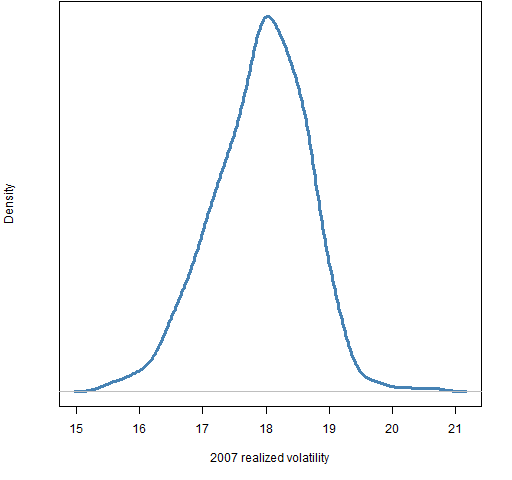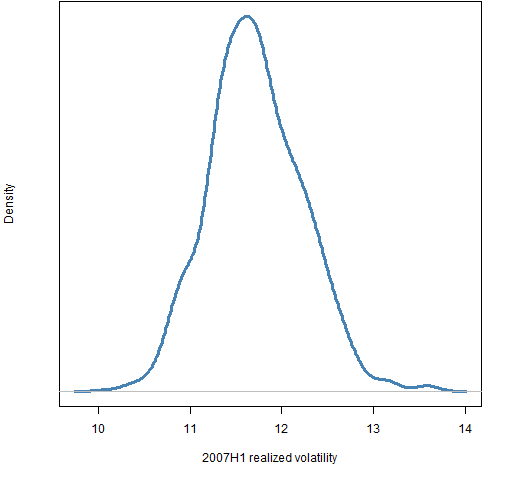## Volatility and tracking error constraints

Generate random portfolios with restrictions on volatility or tracking error.

## Preparation

This presumes that you can do basic random portfolio generation.  For example, that you have mastered “Very simple long-only”.

• Portfolio Probe

You need to have the Portfolio Probe package loaded into your R session:

`require(PortfolioProbe)`

If you don’t have Portfolio Probe, see “Demo or Buy”.

### Doing the example

`require(pprobeData)`

## Doing it

We generate random portfolios with the following constraints:

• maximum volatility
• volatility within a range
• maximum tracking error
• tracking error within a range

### maximum volatility

We generate 1000 random portfolios with volatility at most 12%:

```rpVolMax <- random.portfolio(1000, priceVector,
long.only=TRUE, gross=grossVal, variance=xaLWvar06,
existing=curPortfol, var.constraint=.12^2/252)```

We need to transform the 12% volatility into the scale of our variance matrix, which is daily.

### volatility within a range

To impose a range on volatility, you need to give a two-column matrix as the `var.constraint` argument.  Here we constrain the volatility to be between 11.9% and 12%:

```rpVolRange <- random.portfolio(1000, priceVector,
long.only=TRUE, gross=grossVal, variance=xaLWvar06,
existing=curPortfol,
var.constraint=rbind(c(.119, .12)^2/252))```

The  value given to `var.constraint` looks like:

```> rbind(c(.119, .12)^2/252)
[,1]         [,2]
[1,] 5.619444e-05 5.714286e-05```

You could get the same thing with:

```> t(as.matrix(c(.119, .12)^2/252))
[,1]         [,2]
[1,] 5.619444e-05 5.714286e-05```

### maximum tracking error

Tracking error is constrained with the `bench.constraint` argument.  But this argument is on the variance scale — that is, some scaling of the squared tracking error.

The variance we are using is from daily returns.  We want 1000 portfolios that have at most 2% (predicted) tracking error.

```rpTEmax <- random.portfolio(1000, priceVector,
long.only=TRUE, gross=grossVal,
variance=xaLWvar06EqWt, existing=curPortfol,
bench.constraint=c(EqWt=.02^2/252))```

There must be a name on the value given to `bench.constraint` so that it knows which asset to use as the benchmark.

### tracking error within a range

We generate 1000 random portfolios with predicted tracking error that is between 3.5% and 4%:

```rpTErange <- random.portfolio(1000, priceVector,
long.only=TRUE, gross=grossVal,
variance=xaLWvar06EqWt, existing=curPortfol,
bench.constraint=rbind(EqWt=c(.035, .04)^2/252))```

The value given to `bench.constraint` in this case is a matrix with one named row and two columns:

```> rbind(EqWt=c(.035, .04)^2/252)
[,1]         [,2]
EqWt 4.861111e-06 6.349206e-06```

## Explanation

The `var.constraint` argument constrains the variance.  If it is given one number, then that is taken to be the maximum value allowed.  To specify a range, you give a two-column matrix where the first column gives the minimum allowed, and the second column gives the maximum allowed.  (A vector of values would be maximums for multiple variances.)

The `bench.constraint` argument takes values just like `var.constraint` except that the value or the row needs to be named with the identifier of the benchmark.

## Further details

It is possible to constrain both volatility and tracking error.  Here we constrain volatility to be between 11% and 12% and the tracking error to be 3.5% to 4%:

```rpVolTErange <- random.portfolio(1000, priceVector,
long.only=TRUE, gross=grossVal,
variance=xaLWvar06EqWt, existing=curPortfol,
bench.constraint=rbind(EqWt=c(.035, .04)^2/252),
var.constraint=rbind(c(.11, .12)^2/252))```

Remember that since we are using argument names, the order of the arguments doesn’t matter (except in this case the number of portfolios to generate and the prices).

#### predicted volatility

We can check that the volatility is really being restricted by collecting the ex-ante variances for the portfolios:

```volCheck <- sqrt(252 * unlist(randport.eval(rpVolMax,
keep='var.values')))```

The `volCheck` object holds the predicted volatility for each portfolio.  The `randport.eval` function gets the answer that the optimizer would have if it arrived at the random portfolio.  You then have the option to keep only some components of the answer (or to apply a function to it).  In this case we are keeping only the predicted variances.

We can look at the achieved volatilities:

```> summary(volCheck)
Min. 1st Qu.  Median    Mean 3rd Qu.    Max.
0.08973 0.11150 0.11610 0.11430 0.11880 0.12000
> tail(sort(volCheck))
var.values.V0 var.values.V0 var.values.V0
0.1199847     0.1199902     0.1199902
var.values.V0 var.values.V0 var.values.V0
0.1199926     0.1199931     0.1199993```

This exercise would be slightly more complicated for the portfolios that constrain tracking error because in that case there are two values in the `var.values` component: the portfolio variance and the variance relative to the benchmark.

#### realized volatility

We can use the moves in “Returns and realized volatility” to get the realized volatility for the subsequent year and then plot the distribution.

```retVolMax07 <- valuation(rpVolMax,
xassetPrices[251:502,], returns="log")
volVolMax07 <- apply(retVolMax07, 2, sd) *
sqrt(252) * 100
plot(density(volVolMax07)) # essentially Figure 1```

Figure 1: Volatility realized in 2007 for the `rpVolMax` portfolios.The realized volatility for all of the portfolios is significantly bigger than 12%.  However, that need not mean we have done anything wrong because 2007 is when volatility started to heat up.  We can look at the realized volatility in the first half of 2007 when volatility was still low.

```retVolMax07H1 <- valuation(rpVolMax,
xassetPrices[251:376,], returns="log")
volVolMax07H1 <- apply(retVolMax07H1, 2, sd) *
sqrt(252) * 100```

Figure 2: Volatility realized in H1 of 2007 for the `rpVolMax` portfolios.This is a more reassuring picture.

## Troubleshooting

• Are you giving `var.constraint` or `bench.constraint` a value (or values) that correspond to the variance matrix that you are using?  Is the variance for returns or percent returns?  What is the time frame of the returns?
• If you are wanting a range for the volatility or tracking error, are you giving a two-column matrix?